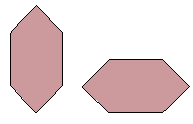Email us to get an instant 20% discount on highly effective K-12 Math & English kwizNET Programs!

#### Online Quiz (WorksheetABCD)

Questions Per Quiz = 2 4 6 8 10

### MEAP Preparation - Grade 7 Mathematics1.145 Geometry Part Three - Review Test

 Q 1: The two figures aresimilar and not congruent1similar and congruent Q 2: The formula for volume of a cube islength x widthlength + width + heightlength x width x height Q 3: Two figures that are exactly the same shape and size are said to becongruentdifferentsimilar Q 4: What is the volume of a cube with length 3 cm27 cubic cm18 cubic cm9 cubic cm Q 5: What is the perimeter of a rectangle with length 120 m and width 2 m?240 sq m200 m244 m122 m Q 6: The two figures arenot congruentnot similar Q 7: In a symmetrical figure the folded line is calledthe line of symmetryradiusdiameter Q 8: Are these figures congruent?NoYes Question 9: This question is available to subscribers only! Question 10: This question is available to subscribers only!